# Irodov Solutions: Elastic Deformations of a Solid Body - 1 Notes | Study I. E. Irodov Solutions for Physics Class 11 & Class 12 - JEE

## JEE: Irodov Solutions: Elastic Deformations of a Solid Body - 1 Notes | Study I. E. Irodov Solutions for Physics Class 11 & Class 12 - JEE

The document Irodov Solutions: Elastic Deformations of a Solid Body - 1 Notes | Study I. E. Irodov Solutions for Physics Class 11 & Class 12 - JEE is a part of the JEE Course I. E. Irodov Solutions for Physics Class 11 & Class 12.
All you need of JEE at this link: JEE

Q. 290. What pressure has to be applied to the ends of a steel cylinder to keep its length constant on raising its temperature by 100°C?

Solution. 290. Variation of length with temperature is given by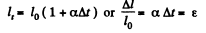But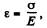Thus σ = αΔtE, which is the sought stress of pressure.
Putting the value of a and E from Appendix and taking Δt = 100°C, we get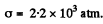Q. 291. What internal pressure (in the absence of an external pressure) can be sustained
(a) by a glass tube;
(b) by a glass spherical flask, if in both cases the wall thickness is equal to Δr = 1.0 mm and the radius of the tube and the flask equals r = 25 mm?

Solution. 291. (a) Consider a transverse section of the tube and concentrate on an element which subtends an angle Δφ at the centre. The forces acting on a portion of length A/ on the element are
(1) tensile forces side ways of magnitude σΔrΔl.
The resultant of these is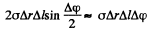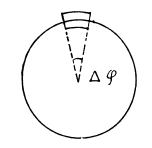(2) The force due to fluid pressure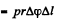Since these balance, we get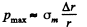where σm is the maximum tensile force. Putting the values we get  atmos.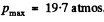(b) Consider an element of area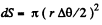about z -a x is chosen arbitrarily. There are tangential tensile forces all around the ring of the cap. Their resultant is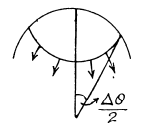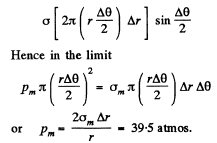Q. 292. A horizontally oriented copper rod of length l = 1.0 m is rotated about a vertical axis passing through its middle. What is the number of rps at which this rod ruptures?

Solution. 292. Let us consider an element of rod at a distance x from its rotation axis (Fig.). From Newton’s second law in projection form directed towards the rotation axis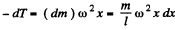On integrating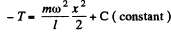But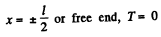Thus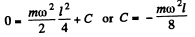Hence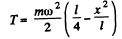Thus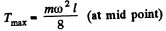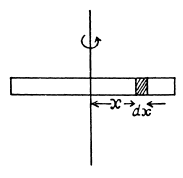Condition renuired for the. problem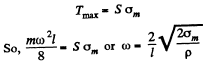Hence the sought number of r p s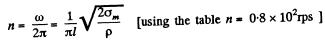Q. 293. A ring of radius r = 25 cm made of lead wire is rotated about a stationary vertical axis passing through its centre and perpendicular to the plane of the ring. What is the number of rps at which the ring ruptures?

Solution. 293. Let us consider an element of the ring (Fig.). From Newton’s law Fn = mwn for this element, we get,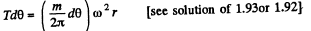So,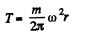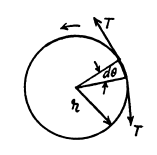Condition for the problem is :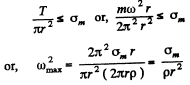Thus sought number of rps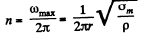Using the table of appendices n = 23rps

Q. 294. A steel wire of diameter d = 1.0 mm is stretched horizontally between two clamps located at the distance l = 2.0 m from each other. A weight of mass m = 0.25 kg is suspended from the midpoint O of the wire. What will the resulting descent of the point O be in centimetres?

Solution. 294.  Let the point O desend by the distance x (Fig.). From the condition of equilibrium of point O.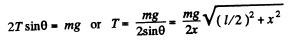(1)

Now,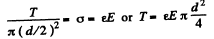(2)

(σ here is stress and ε is strain.)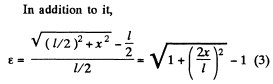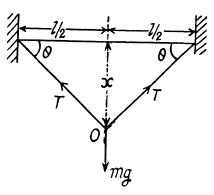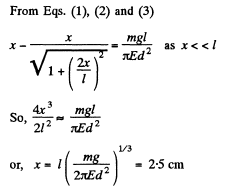Q. 295. A uniform elastic plank moves over a smooth horizontal plane due to a constant force F0 distributed uniformly over the end face. The surface of the end face is equal to S, and Young's modulus of the material to E. Find the compressive strain of the plank in the direction of the acting force.

Solution. 295. Let us consider an element of the rod at a distance x from the free end (Fig.). For the considered element ‘T - T’ are internal restoring forces which produce elongation and dT provides the acceleration to the element. For the element from Newton’s law :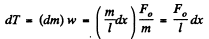As free end has zero tension, on integrating the above expression,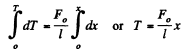Elongation in the considered element of lenght dx :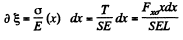Thus total elengation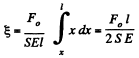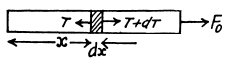Hence the sought strain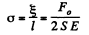Q. 296. A thin uniform copper rod of length l and mass m rotates uniformly with an angular velocity ω in a horizontal plane about a vertical axis passing through one of its ends. Determine the tension in the rod as a function of the distance r from the rotation axis. Find the elongation of the rod.

Solution. 296. Let us consider an element of the rod at a distance r from it’s rotation axis. As the element rotates in a horizontal circle of radius r, we have from Newton’s second law in projection form directed toward the axis of rotation :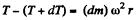or,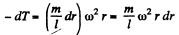At the free end tension becomes zero. Integrating the above experession we get, thus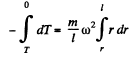Thus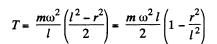Elongation in elemental length dr is given by :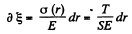(where S is the cross sectional area of the rod and T is the tension in the rod at the considered element)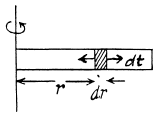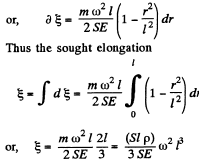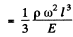(where p is the density of the copper.)

Q. 297. A solid copper cylinder of length l = 65 cm is placed on a horizontal surface and subjected to a vertical compressive force F = 1000 N directed downward and distributed uniformly over the end face. What will be the resulting change of the volume of the cylinder in cubic millimetres?

Solution. 297. Volume of a solid cylinder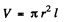So,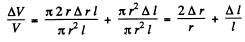(1)
But longitudinal strain Δl/l and accompanying lateral strain Δ r/r are related as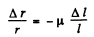(2)

Using (2) in (1), we get :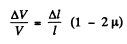(3)

But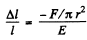(Because the increment in the length of cylinder Δl is negative)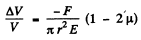Thus,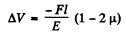Negative sign means that the volume of the cylinder has decreased.

Q. 298. A copper rod of length l is suspended from the ceiling by one of its ends. Find:
(a) the elongation Δl of the rod due to its own weight;
(b) the relative increment of its volume ΔVIV.

Solution. 298. (a) As free end has zero tension, thus the tension in the rod at a vestical distance y from its lower end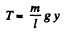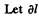be the elongation of the element of length dy, then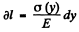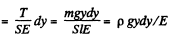(where p is the density of the copper)

Thus the sought elongation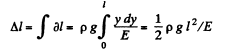(2)

(b) If the longitudinal (tensile) strain is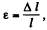the accompanying lateral (compressive) strain is given by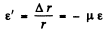(3)

Then since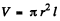we have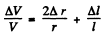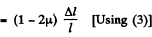where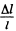is given in part (a), μ is the Poisson ratio for copper.

Q. 299. A bar made of material whose Young's modulus is equal to E and Poisson's ratio to μ, is subjected to the hydrostatic pressure p. Find:
(a) the fractional decrement of its volume;
(b) the relationship between the compressibility β and the elastic constants E and μ.

Solution. 299.  Consider a cube of unit length before pressure is applied. The pressure acts on each face. The pressures on the opposite faces constitute a tensile stress producing longitudianl compression and lateral extension. The compressions is P/E and the lateral extension is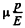The net result is a compression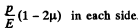Hence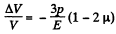because from symmetry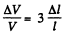(b) Let us consider a cube under an equal compressive stress σ, acting on all its faces.

Then,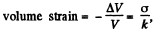(1)

where k is the bulk modulus of elasticity.

So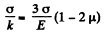or,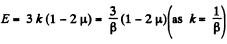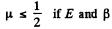are both to remain positive.

Q. 300. One end of a steel rectangular girder is embedded into a wall (Fig. 1.74). Due to gravity it sags slightly. Find the radius of curvature of the neutral layer (see the dotted line in the figure) in the vicinity of the point O if the length of the protruding section of the girder is equal to l = 6.0 m and the thickness of the girder equals h = 10 cm.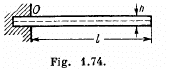Solution. 300. A beam clamped at one end and supporting an applied load at the free end is called a cantilever. The theory of cantilevers is discussed in advanced text book on mechanics. The key result is that elastic forces in the beam generate a couple, whose moment, called the moment of resistances, balances the external bending moment due to weight of the beam, load etc. The moment of resistance, also called internal bending moment (I.B.M) is given by

I.B.M. = EI/R

Here R is the radius of curvature of the beam at the representative point (x, y). I is called the geometrical moment of inertia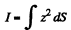of the cross section relative to the axis passing through the netural layer which remains unstretched. (Fig.l.). The section of the beam beyond P exerts the bending moment N (x) and we have,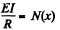If there is no load other than that due to the weight of the beam, then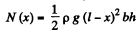where p = density of steel. Hence, at x = 0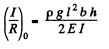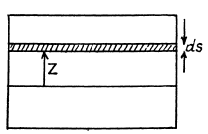Here b = width of the beam perpendicular to paper.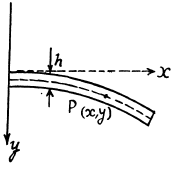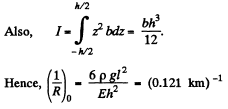Q. 301. The bending of an elastic rod is described by the elastic curve passing through centres of gravity of rod's cross-sections. At small bendings the equation of this curve takes the form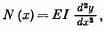where N (x) is the bending moment of the elastic forces in the cross- section corresponding to the x coordinate, E is Young's modulus, I is the moment of inertia of the cross-section relative to the axis pass- ing through the neutral laye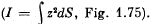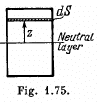Suppose one end of a steel rod of a square cross-section with side a is embedded into a wall, the protruding section being of length l (Fig. 1.76). Assuming the mass of the rod to be negligible, find the shape of the elastic curve and the deflection of the rod X, if its end A experiences
(a) the bending moment of the couple N0;
(b) a force F oriented along the y axis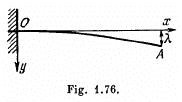Solution. 301. We use the equation given above and use the result that when y is small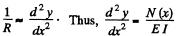(a) Here N (x) = N0 is a constant. Then integration gives,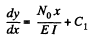But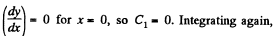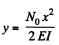where we have used y = 0 for x = 0 to set the constant of integration at zero. This is the equation of a parabola. The sag of the free end is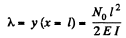(b) In this case N (x) - F (l - x) because the load F at the extremity is balanced by a similar force at F directed upward and they constitute a couple. Then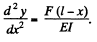Integrating,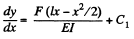As before C1 = 0. Integrating again, using y = 0 for x = 0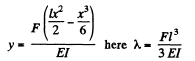Here for a square cross section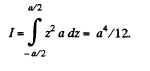The document Irodov Solutions: Elastic Deformations of a Solid Body - 1 Notes | Study I. E. Irodov Solutions for Physics Class 11 & Class 12 - JEE is a part of the JEE Course I. E. Irodov Solutions for Physics Class 11 & Class 12.
All you need of JEE at this link: JEEUse Code STAYHOME200 and get INR 200 additional OFF

## I. E. Irodov Solutions for Physics Class 11 & Class 12

111 docs

Track your progress, build streaks, highlight & save important lessons and more!

,

,

,

,

,

,

,

,

,

,

,

,

,

,

,

,

,

,

,

,

,

;